Aptitude Tests 4 Me

Non Verbal Logic

Detailed solution

77.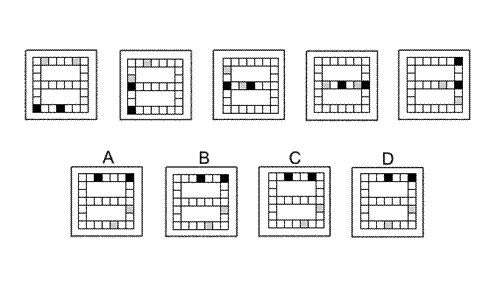Solution: B Explanation: In this question there is a grid in the shape of a number 8. There are two grey squares that start in the top row of the grid, moving three places at a time, and following a path resembling a letter S. There are two black squares that start in the bottom row of the grid, moving three places at a time, and following a shape resembling a backwards letter S. When these rules are applied, the next diagram of the sequence is B.

78.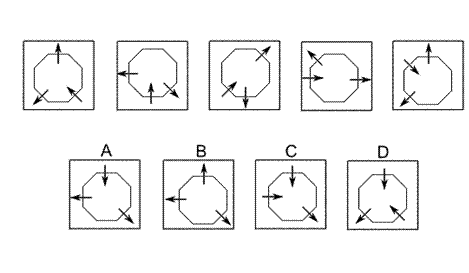Solution: A Explanation: In this question there is an octagon with arrows perpendicular to three of its sides - two arrows point away from the centre of the octagon; the other arrow points towards the centre. The two arrows pointing away from the centre rotate about the centre of the octagon each time by 90 degree anticlockwise. The one arrow pointing towards the centre rotates about the centre of the octagon by 45 degree clockwise each time. When these rules are applied simultaneously, the correct answer is A.

79.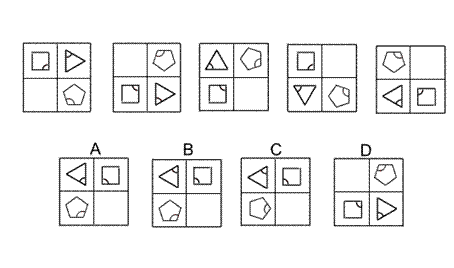Solution: B Explanation: In this question there are two reflections that are applied alternately. The first is a reflection in the horizontal axis. The second is a reflection in the diagonal stretching from the bottom left corner to the top right corner. To obtain the next diagram of the sequence the first reflection should be applied next, and the correct answer is B.

80.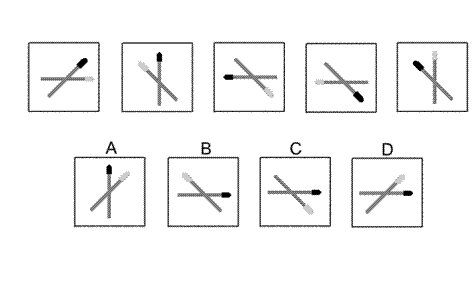81.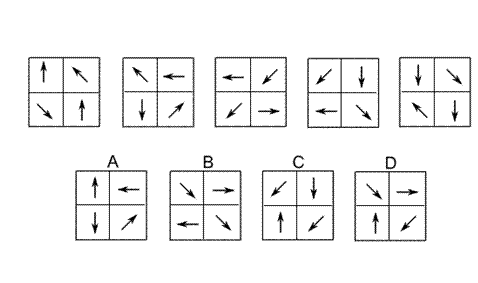Solution: D Explanation: In this question the two arrows in the top row rotate by 45 degree anticlockwise each time and the two arrows in the bottom row rotate by 45 degree clockwise each time. Following these rules, the correct answer is D.

82.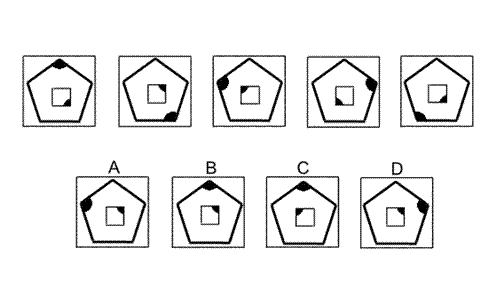Solution: B Explanation: In this question there is a square inside a regular polygon. Each shape follows its own rule. The square rotates by 90 degree anticlockwise each time. Following this rule, the next diagram of the sequence could be A, B or D. The pentagon rotates by 144 degree clockwise each time. When this rule is also applied, the next diagram of the sequence must be B.

83.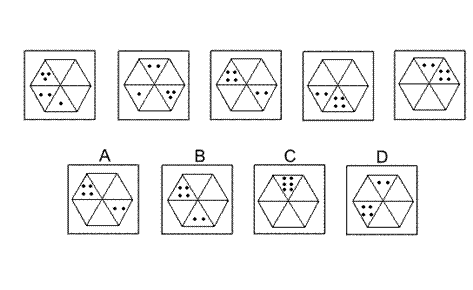Solution: A Explanation: In this question the dots move clockwise around the six triangles of the hexagon, moving a number of places equal to the number of dots. When two sets of dots land on the same triangle, their scores are added together. To obtain the next diagram of the sequence, therefore, the four dots should move four places clockwise and the two dots should move two places clockwise, and the correct answer is A.

84.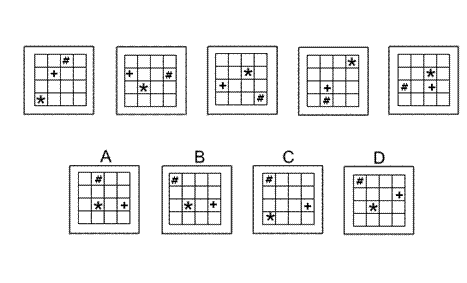Solution: B Explanation: In this question there is an asterisk, a hash sign and a plus sign. Each shape moves around the grid according to its own rule. The asterisk moves up and down the diagonal stretching from the bottom left of the grid to the top right of the grid, moving one place each time. When this rule is applied, the correct answer could be A, B or D. The hash sign moves around the 12 squares around the perimeter of the grid, moving two squares clockwise each time. When this rule is also applied, the correct answer could be B or D. The plus sign moves around the 8 squares of the middle two rows of the grid, moving one place anticlockwise each time. When this rule is also applied, the correct answer must be B.

85.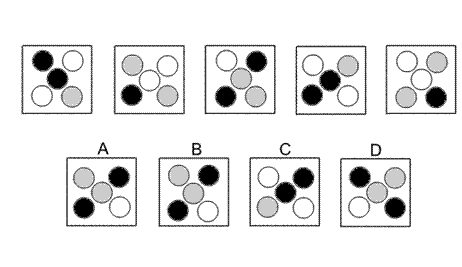Solution: D Explanation: In this question there are five coloured circles that rotate each time by 90 degree clockwise. When this rule is applied, the correct answer could be A, B or D. At the same time the colours change from one diagram to the next - black changes to white, white changes to grey and grey changes to black. When this rule is also applied, the correct answer must be D.

 1 2 3 4 5 6 7 8 9 10 11 12 13 14 15 16 17 18 19 20 21 22 23 24 25 26 27 28 29 30 31 32 33 34 35 36 37 38 39 40 41 42 43 44 45 46 47 48 49 50 51 52 53 54 55 56 57 58 59 60 61 62 63 64 65 66 67 68 69 70 71 72 73 74 75 76 77 78 79 80 81 82 83 84 85 86 87 88Passage ReadingVerbal LogicNon Verbal LogicNumerical LogicData InterpretationReasoningAnalytical AbilityBasic NumeracyAbout UsContactPrivacy PolicyMajor TestsFAQ